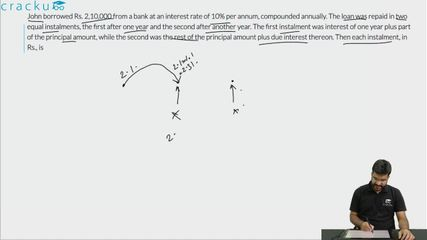Question 79

# John borrowed Rs. 2,10,000 from a bank at an interest rate of 10% per annum, compounded annually. The loan was repaid in two equal instalments, the first after one year and the second after another year. The first instalment was interest of one year plus part of the principal amount, while the second was the rest of the principal amount plus due interest thereon. Then each instalment, in Rs., is

Solution

We have to equate the installments and the amount due either at the time of borrowing or at the time when the entire loan is repaid. Let us bring all values to the time frame in which all the dues get settled, i.e, by the end of 2 years.

John borrowed Rs. 2,10,000 from the bank at 10% per annum. This loan will amount to 2,10,000*1.1*1.1 = Rs.2,54,100 by the end of 2 years.
Let the amount paid as installment every year be Rs.x.

John would pay the first installment by the end of the first year. Therefore, we have to calculate the interest on this amount from the end of the first year to the end of the second year. The loan will get settled the moment the second installment is paid.

=> 1.1x + x = 2,54,100
2.1x = 2,54,100
=> x = Rs. 1,21,000.

Therefore, 121000 is the correct answer.

### View Video Solution• All Quant CAT Formulas and shortcuts PDF
• 30+ CAT previous papers with solutions PDF